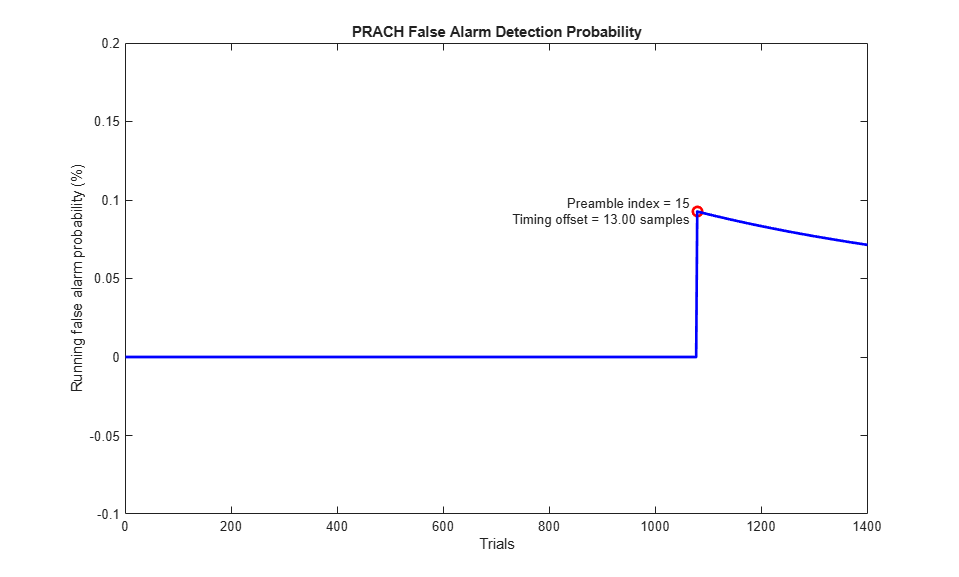Documentation

## PRACH False Alarm Probability Conformance Test

This example shows how the LTE Toolbox™ can be used to model a TS36.104 Physical Random Access Channel (PRACH) false alarm probability conformance test. In this case the probability of erroneous detection of a PRACH preamble is to be measured when the input to the PRACH detector is only noise.

### Introduction

Measuring the probability of erroneous detection is defined in TS36.104, Section 8.4.1 [ 1 ]. The target defined for any bandwidth, all frame structures and for any number of receive antennas is a false alarm probability less than or equal to 0.1%.

The input to the PRACH detector is made up exclusively of a Gaussian noise signal. Detection is attempted with all possible cell preamble indices, and if a preamble is detected within the noise, a false detection is accumulated.

### UE Configuration

A configuration structure for the User Equipment (UE) is created and set to use 6 resource blocks in Frequency Division Duplexing (FDD).

```ue.NULRB = 6; % Number of resource blocks ue.DuplexMode = 'FDD'; % FDD duplexing mode ```

### PRACH Configuration

Setup the PRACH configuration structure. The standard documents do not specify any values since no PRACH is transmitted.

```prach.Format = 0; % Preamble format prach.SeqIdx = 2; % Logical root sequence index prach.CyclicShiftIdx = 1; % Cyclic shift configuration index prach.HighSpeed = 0; % High speed flag prach.FreqOffset = 0; % Use default frequency resource index prach.PreambleIdx = []; % Empty since no preamble is transmitted ```

### Establish PRACH Generator Output Length for This Configuration

ltePRACHInfo returns PRACH dimensionality information given UE-specific settings and PRACH channel transmission configuration. From this information the PRACH output length can be calculated using the PRACH duration in subframes, the duration of one subframe in seconds (1ms) and the PRACH modulator sampling rate.

```info = ltePRACHInfo(ue, prach); nSamples = info.SamplingRate*info.TotSubframes*0.001; ```

### Loop for Detection in Each Subframe

Initialize the false detection count and enter a loop to process multiple detection trials. For each detection trial a Gaussian noise signal (of length `nSamples`) is created and detection is performed on it for every possible cell preamble index using ltePRACHDetect. In the ideal case, no preambles should have been detected. In the case where a preamble has been detected, a false alarm for this trial is recorded.

```numTrials = 1400; falseCount = 0; % Initialize false detection counter rng('default'); % Random number generator to default state runningP=zeros(1, numTrials); for nt = 1:numTrials % Create noise noise = complex(randn(nSamples, 1), randn(nSamples, 1)); % Attempt detection for all cell preamble indices (0...63) [detected,offset] = ltePRACHDetect(ue, prach, noise, 0:63); % Record false alarm if (~isempty(detected)) falseCount = falseCount+1; end % Calculate running false alarm probability runningP(nt) = falseCount/nt*100; % Plot information about false alarm (if applicable) if (~isempty(detected)) plot(nt,runningP(nt),'ro','LineWidth',2,'MarkerSize',7); hold on; text(nt,runningP(nt), sprintf(['Preamble index = %d' ... ' \nTiming offset = %0.2f samples '],detected,offset), ... 'HorizontalAlignment','right'); end end ```### Compute Final False Alarm Probability

Compute the final false alarm probability and plot the running false alarm probability across the simulation.

```P = falseCount / numTrials; plot(1:numTrials,runningP,'b','LineWidth',2); axis([0 numTrials+1 -0.1 0.2]); xlabel('Trials'); ylabel('Running false alarm probability (%)'); title('PRACH False Alarm Detection Probability'); fprintf('\nFalse alarm probability = %0.4f%%',P*100); ```
`False alarm probability = 0.0714%`### Selected Bibliography

1. 3GPP TS 36.104 "Base Station (BS) radio transmission and reception"